# Other way to look at rotation

## Roll, Pitch, Yaw

### YAW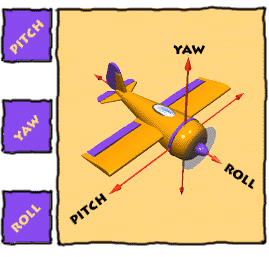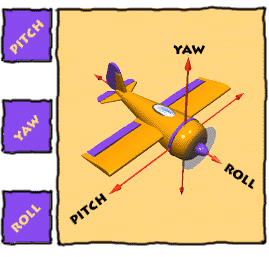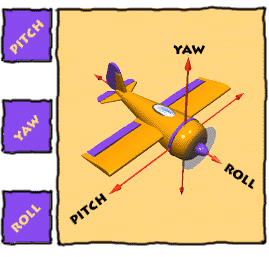#### Rotation around the vertical axis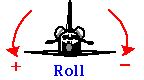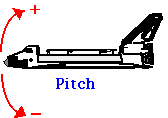## Euler Angles

written by Ian Humphrey <I.Humphrey@sct.gu.edu.au>

The Euler Angles (a, b, g) comprise a parametrisation of arbitrary rotations in three-dimensional space.
The description in this document coincides with that of "Angular Momentum in Quantum Mechanics" by Edmonds and "Angular Momentum" by Brink and Satchler, two standard texts in the field.

The effect of Euler angle rotation R=(a, b, g) is briefly as follows:
1. We start in frame S with cartesian axes, x, y and z.
2. We begin with a positive (anti-clockwise) rotation of magnitude a about the z axis of S. The resulting frame is called S'.
3. Next a positive rotation of magnitude b about the y' axis of frame S'. The resulting frame is called S''.
4. Finally a positive rotation of magnitude g about the z'' axis of S''. The resulting frame is called S'''.

### STEP 3: Rotation of g about z''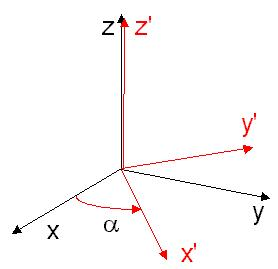## Quaternions.

 Web www.vrarchitect.net